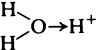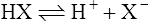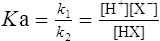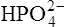Chapter 3

# The molecular environment

## Hydrogen ions and the idea of acidity

There is something special about hydrogen ions which distinguishes them from all other ions and which has long been recognized in the chemical idea of ‘acidity’. Essentially, the hydrogen ion is a proton, i.e. a hydrogen nucleus that has lost its orbital electron, and exists in aqueous solution in the hydrated form, H3O+ formed by the water oxygen donating a lone pair of electrons to the proton

I=12icizi2This hydrated form is sometimes referred to as a ‘hydronium’ or ‘oxonium’ ion but, provided it is understood that aqueous solutions are under consideration, the term ‘hydrogen ion’ (H+) is less confusing. In one respect hydrogen ions in solution seem to retain the characteristics of subatomic smallness and mobility associated with the proton; they are subject to two distinct transfer processes: (1) by diffusion like all other ions and (2) by transfer between adjacent molecules, often water molecules, by a mechanism which, though not completely understood, probably accounts for their high mobility.

An acid was defined by Bronsted in 1923 as a molecule or ion with a tendency to give up protons in solution and a base as a molecule or ion which has a tendency to acquire protons and thereby form an acid. There is a complementary relationship between conjugate pairs of acid and base. Thus considering the equilibrium

HXH++X (3·1)(3·1)

HX is an acid, since on ionization it loses a proton, whereas the ion X is a base since it has a tendency to acquire a proton and form HX. HX is the conjugate acid to base X and conversely X is the conjugate base to acid HX.

Different acids vary considerably in their strength. A strong acid is one that gives up protons readily whereas a weak acid gives them up reluctantly. A strong base acquires protons avidly and a weak base less readily. Strong acids have relatively weak conjugate bases (i.e. when the equilibrium of Equation (3·1) lies to the right), whereas weak acids have strong conjugate bases (equilibrium lying to the left).

The strength of an acid can be expressed as its dissociation constant, Ka, by applying the law of mass action* to the equilibrium of Equation (3·1). Thus the velocity of the reaction from left to right (V1) is given by the equation

V1=k1[HX] (3·2)(3·2)

where k1 is the velocity coefficient for the forward reaction and [HX] is the ‘activity’ of the acid HX which for dilute solutions, such as occur in living organisms, can to all intents and purposes be considered as equal to the concentration of the acid. Considering the reverse reaction, its velocity (V2) is given by

V2=k2[H+][X] (3·3)(3·3)

where k2 is the velocity coefficient of the reaction from right to left and [H+] and [X] are the concentrations of hydrogen ions and the conjugate base respectively.

k1[HX]=k2[H+][X]by cross multiplying we obtain the definition of the acid dissociation constant Ka:

Ka=k1k2=[H+][X][HX] (3·4)(3·4)

k1 and k2 are both constants so that k1/k2 will also be a constant which is denoted by Ka. For strong acids the concentrations of products [H+] and [X] will be large and the concentration of acid [HX] small so the dissociation constant Ka will be large. Conversely, the dissociation constant of a weak acid will be small.

In Table 3.2 a number of acids of biochemical interest are listed, together with their dissociation constants and conjugate bases. There is a considerable range of acid strengths, represented by the dissociation constants. These range from values greater than unity for the very strong mineral acids to very small values indeed, where Ka involves large negative powers of ten, e.g. for the third ionization of phosphoric acid and for water itself. Examination of the formulae in the first column of the table shows that acids may be either electrically neutral molecules, anions with one or more negative charges (e.g. the ion), zwitterions (such as the zwitterionic form of glycine, NH3+ · CH2 · COO) (page 41) and even cations (such as the ammonium ionwhich is an acid because it has a tendency to lose a proton to form an electrically neutral ammonia molecule). Polybasic acids such as carbonic, citric and orthophosphoric acids have distinct values of Ka for each of their dissociable protons. These dissociation constants may lie close together as in citric acid, where they differ only by a power of ten, or spread far apart as in phosphoric acid, where the Ka values differ by factors of 105. The intermediate ions formed from polybasic acids can behave either as acids or bases according to their environment. Thus the dihydrogen phosphate ionacts as an acid when it loses a proton to form the monohydrogen phosphate ionbut as a base when it gains one to form orthophosphoric acid.

Table 3.2

Only gold members can continue reading. Log In or Register to continue

 Formula of acid Ka pKa Formula of conjugate base HCl Hydrochloric acid >2·9 < −0·46 Cl− CH3·COOH Acetic acid 1·75 × 10−5 4·76 CH3·COO− CH3CH(OH)COOH Lactic acid 8·32 × 10−4 3·08 CH3·CH(OH)COO− C3H5O(COOH)3 Citric acid 9·20 × 10−4 3·04 C3H5O(COOH)2COO− C3H5O(COOH)2COO− Citric acid 2·69 × 10−5 4·57 C3H5O(COOH)(COO−)2 C3H5O(COOH)(COO−)2 Citric acid 1·34 × 10−6 5·87 C3H5O(COO−)3 H2CO3 Carbonic acid 4·31 × 10−7 6·37 HCO3−Carbonic acid 5·61 × 10−11 10·25H3PO4

Dec 10, 2015 | Posted by in General Dentistry | Comments Off on The molecular environment
Premium Wordpress Themes by UFO Themes